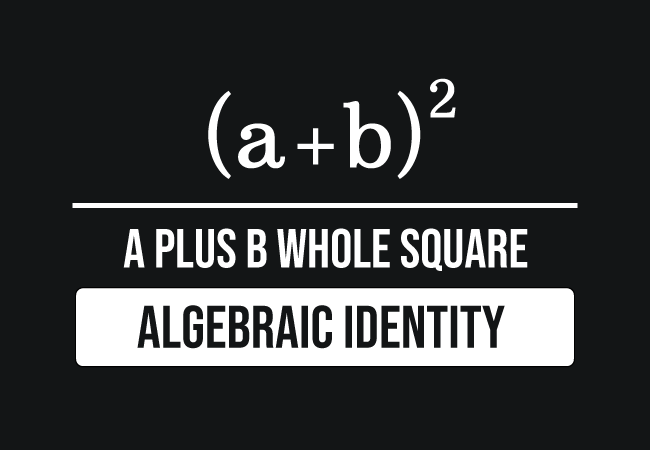# $(a+b)^2$ formula

## Formula

$(a+b)^2 = a^2+b^2+2ab$

### Introduction

When two literals $a$ and $b$ represent two terms in algebraic form. The sum of them is written as $a+b$ in mathematics. It is an algebraic expression and also a binomial. The square of sum of them or binomial is written in mathematics as follows.

$(a+b)^2$The square of sum of two terms is equal to the $a$ squared plus $b$ squared plus $2$ times product of $a$ and $b$.

$(a+b)^2$ $\,=\,$ $a^2+b^2+2ab$

In mathematics, the $a$ plus $b$ whole squared algebraic identity is called in three ways.

1. The square of sum of two terms identity.
2. The square of a binomial rule.
3. The special binomial product formula.

#### Usage

In mathematics, the square of the sum of two terms is used as a formula in two cases.

##### Expansion

The square of the sum of two terms is expanded as the sum of squares of both terms and two times the product of them.

$\implies$ $(a+b)^2 \,=\, a^2+b^2+2ab$

##### Simplification

The sum of squares of the two terms and two times the product of them is simplified as the square of the sum of two terms.

$\implies$ $a^2+b^2+2ab \,=\, (a+b)^2$

##### Examples

$(1) \,\,\,$ Find $(3x+4y)^2$

Now, take $a = 3x$ and $b = 4y$ and substitute them in the expansion of the formula for evaluating its value.

$\implies$ $(3x+4y)^2$ $\,=\,$ $(3x)^2+(4y)^2+2(3x)(4y)$

$\implies$ $(3x+4y)^2$ $\,=\,$ $9x^2+16y^2+2 \times 3x \times 4y$

$\implies$ $(3x+4y)^2$ $\,=\,$ $9x^2+16y^2+24xy$

$(2) \,\,\,$ Simplify $p^2+25q^2+10pq$

$\implies$ $p^2+25q^2+10pq$ $\,=\,$ $p^2+(5q)^2+10pq$

$\implies$ $p^2+25q^2+10pq$ $\,=\,$ $p^2+(5q)^2+2 \times 5 \times p \times q$

$\implies$ $p^2+25q^2+10pq$ $\,=\,$ $p^2+(5q)^2+2 \times p \times 5 \times q$

$\implies$ $p^2+25q^2+10pq$ $\,=\,$ $p^2+(5q)^2+2 \times p \times 5q$

$\implies$ $p^2+25q^2+10pq$ $\,=\,$ $p^2+(5q)^2+2(p)(5q)$

Now, take $a = p$ and $b = 5q$, and simplify the algebraic expression by the $(a+b)^2$ identity

$\implies$ $p^2+25q^2+10pq$ $\,=\,$ $(p+5q)^2$

#### Proofs

The $a$ plus $b$ whole square identity can be derived in mathematics in two different methods.

##### Algebraic method

Learn the algebraic approach to derive the expansion of the $a+b$ whole square formula by the multiplication.

##### Geometric method

Learn the geometric method to derive the expansion of the $a+b$ whole squared identity by the areas of geometric shapes.

A best free mathematics education website that helps students, teachers and researchers.

###### Maths Topics

Learn each topic of the mathematics easily with understandable proofs and visual animation graphics.

###### Maths Problems

A math help place with list of solved problems with answers and worksheets on every concept for your practice.

Learn solutions

###### Subscribe us

You can get the latest updates from us by following to our official page of Math Doubts in one of your favourite social media sites.